Category: math

My Google+ account is going away on April 2, 2019, and all attempts to automatically backup my G+ posts seem to fail so far. So i’ll try to rescue here some of them, in chronological order and around one theme. Today, Grothendieck-stuff, part one.

May 30th, 2013

Recordings of a 1972 talk by Grothendieck at Cern “Réflexions sur la science- responsabilité du savant”.
If you don’t have time to listen to all 138 minutes, try to grab from part1 the fragment 29:10 – 30:40 on “the strange ritual of inviting experts to give a talk on some esoteric subject for an audience of 50 to 100 people, one or two of whom will perhaps be able to painfully understand a few bits and pieces, and all others find themselves in a position of humiliation, as they gave in to social pressure to be there, even though the topic itself didn’t interest them at all” (poor translation on my part)
These recordings are illustrative for Grothendieck’s talks in his ‘Survivre’ period, early 70ties.
(h/t Matilde Marcolli on FB)

June 8th, 2013

Grothendieck’s christmas treeIn the pdf-version of “Recoltes et Semailles” Grothendieck writes on page 463 in the Yin-Yang chapter:

“j’ai fini par aboutir à un diagramme, vaguement en forme d’arbre de Noël”

Here’s the actual diagram, from the original typescript of “Les portes sur l’univers”, the appendix to the ‘Clef du Yin et du Yang’.

Sadly, this appendix (and the many drawings contained in it) didn’t make it into the pdf-release of RecS…

June 9th, 2013

Grothendieck’s yin-yang sunflowerGrothendieck’s ‘Les Portes sur l’Univers’ (Gateways to the Universe(?)) is a truly fascinating text, containing several mysterious drawings (and even a bit of icosahedral-math towards the end).

On PU46, he draws the sunflower of yin and yang, having 12 leafs (he claims, corresponding to 12 yin-terms on the inner circle, 12 yang-terms on the outer circle, as well as to the 12 signs of the zodiac…).

He continues: “On l’appellera, au choix, l’accordeon cosmique, ou l’harmonica cosmique, ou (pour mettre tout le monde d’accord) l’harmonium cosmique”.
(One might call it, as one prefers, the cosmic accordion, or the cosmic harmonica, or (in order to seek general consensus) the *cosmic harmony*).

June 10th, 2013

Grothendieck’s icosahedral theorem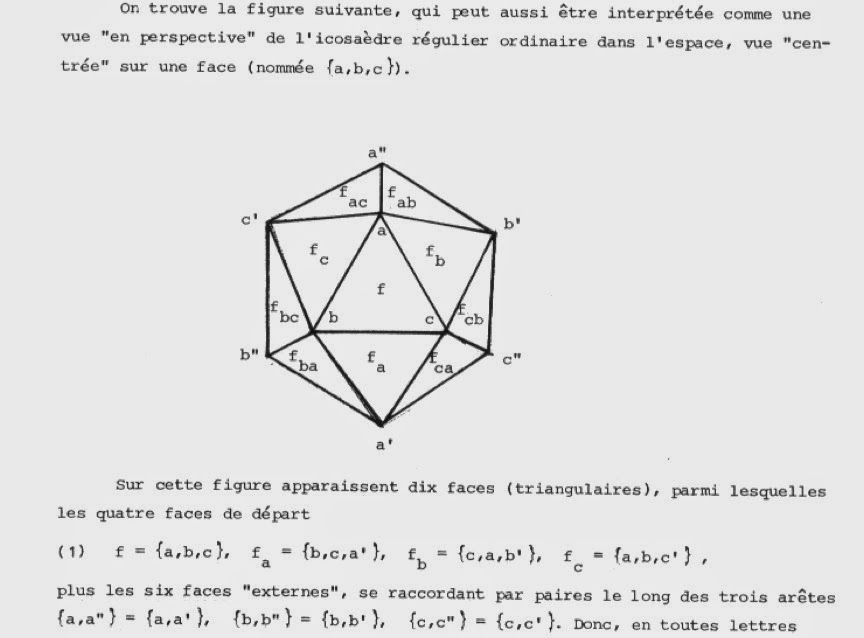On april 12th 1986, Grothendieck decides to add a mathematical annexe to his esoteric text ‘Les portes sur l’univers’.

“Par contre, c’est peu pour mon ardeur de mathématicien, laquelle s’est a nouveau réveillée ces jours derniers – et voila repartie ma réflexion sur l’icosaèdre, cet amour mathématique de mon âge mur! Je vais donc peut-être rajouter a ces notes quelques compléments sur la combinatoire de l’icosaèdre et sur la géométrie des ensembles a six éléments…”

He starts with a set S of 6 elements (the vertices), any pair of elements is an edge and any triple a triangle. He then calls a set of triangles F an *icosahedral structure* provided every edge is contained in exactly two triangles in F.

His main result is that all such icosahedral structures are isomorphic (and has exactly 60 isomorphisms), an icosahedral structure consist of exactly 10 triangles and a choice of triangle determines the structure uniquely. Moreover, there are exactly 12 different such octahedral structures and there is an involution on this set coming from ‘complementary’ structures.

At a first glance, Grothendieck’s result appears to be closely related to one of the surprises in finite group theory: the outer automorphism of the symmetric group on 6 letters.

For more on this and related mathematical mysteries of the octahedron, try:

+John Baez  ‘Some Thoughts on the Number 6’

+Noah Snyder  ‘The Outer Automorphism of S_6’

December 18th, 2013

a chance discovery last month en route from Les Vans – Lablachere (in the Ardeche region), a ‘ferronnerie d’art’ (a wrought-iron workshop) called ‘La Clef des Songes’.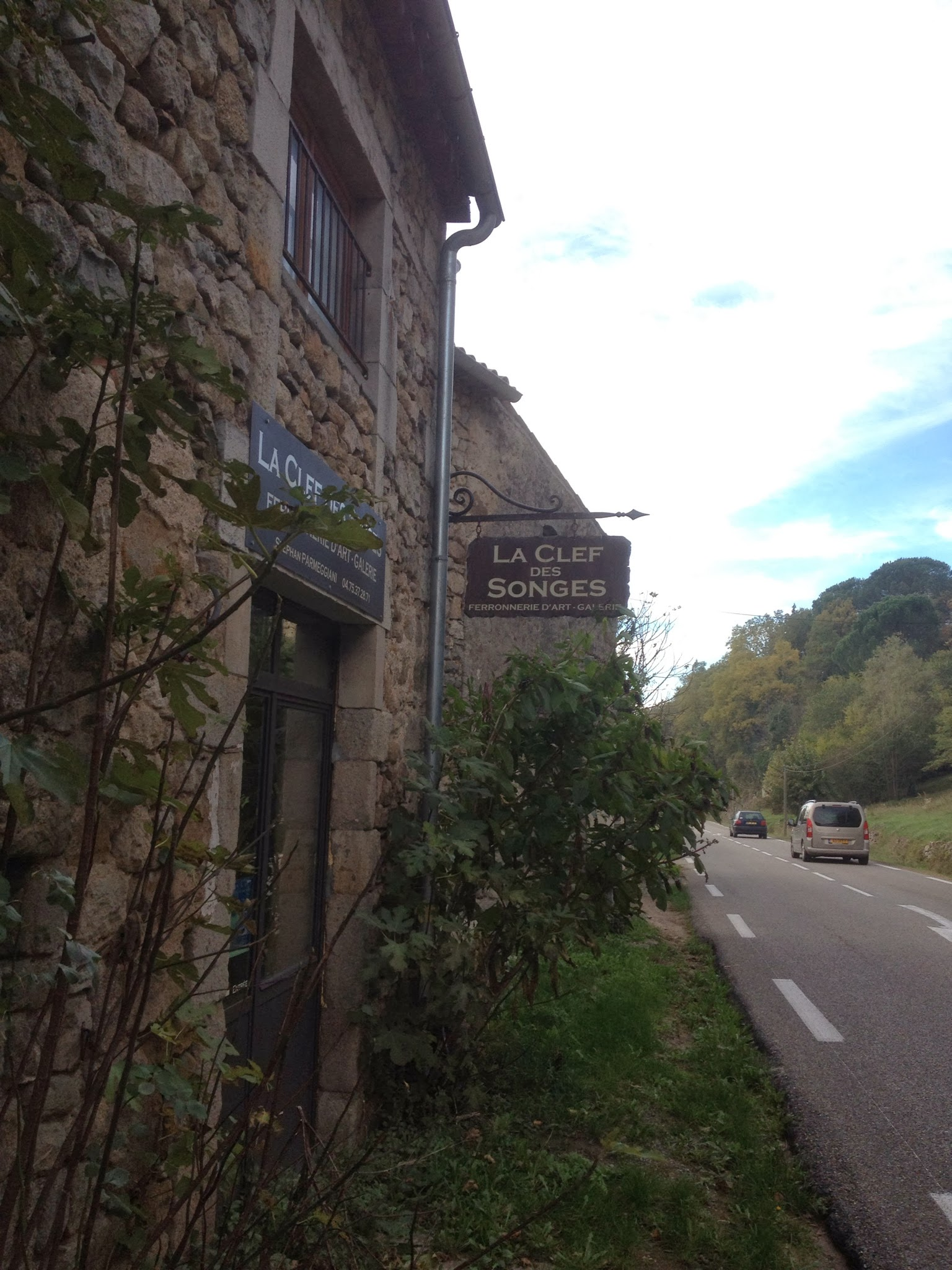All 315 pages of this Grothendieck meditation from 1987 can be found here.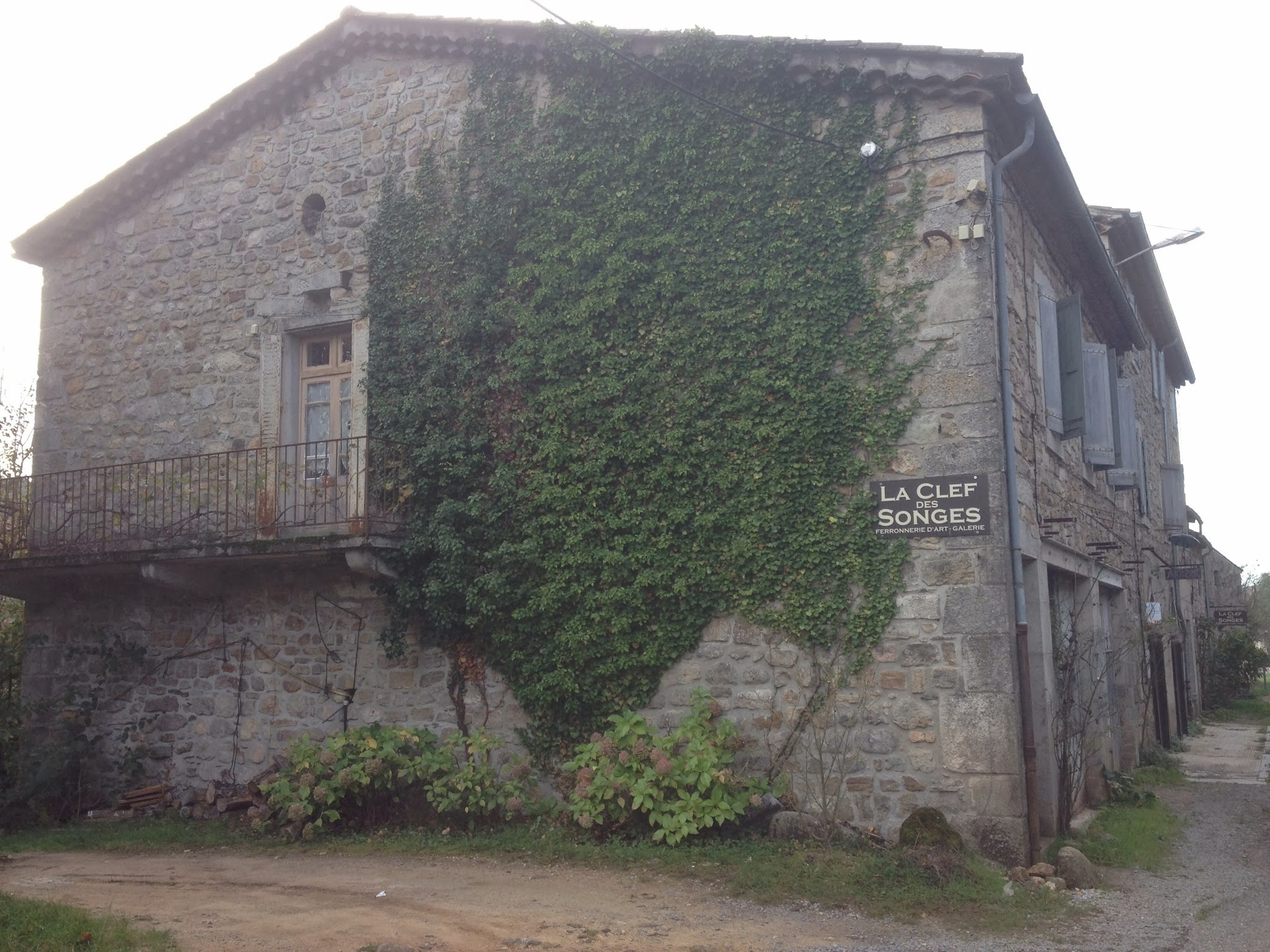The 691 pages of ‘Notes pour la clef des songes’ are a bit harder to get. Fortunately, the mysterious website ‘l’astree’ offers them as a series of 23 pdfs here. Enjoy the read!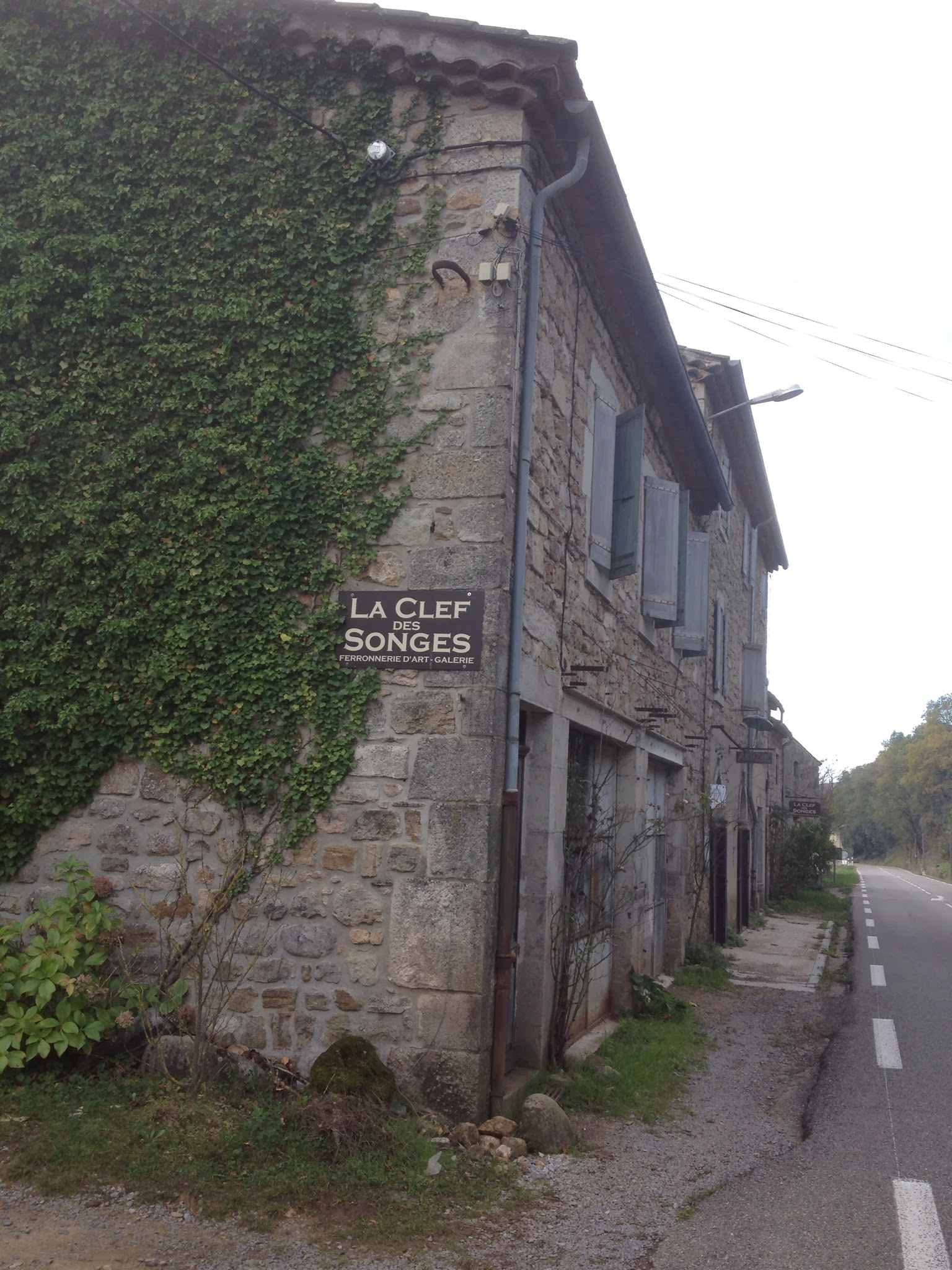January 3rd, 2014

Why did Grothendieck quit mathematics?

After yesterday’s post on the striking similarities between the lives of Grothendieck and JD Salinger it sure felt weird to stumble upon this footnote in “La Clef des Songes”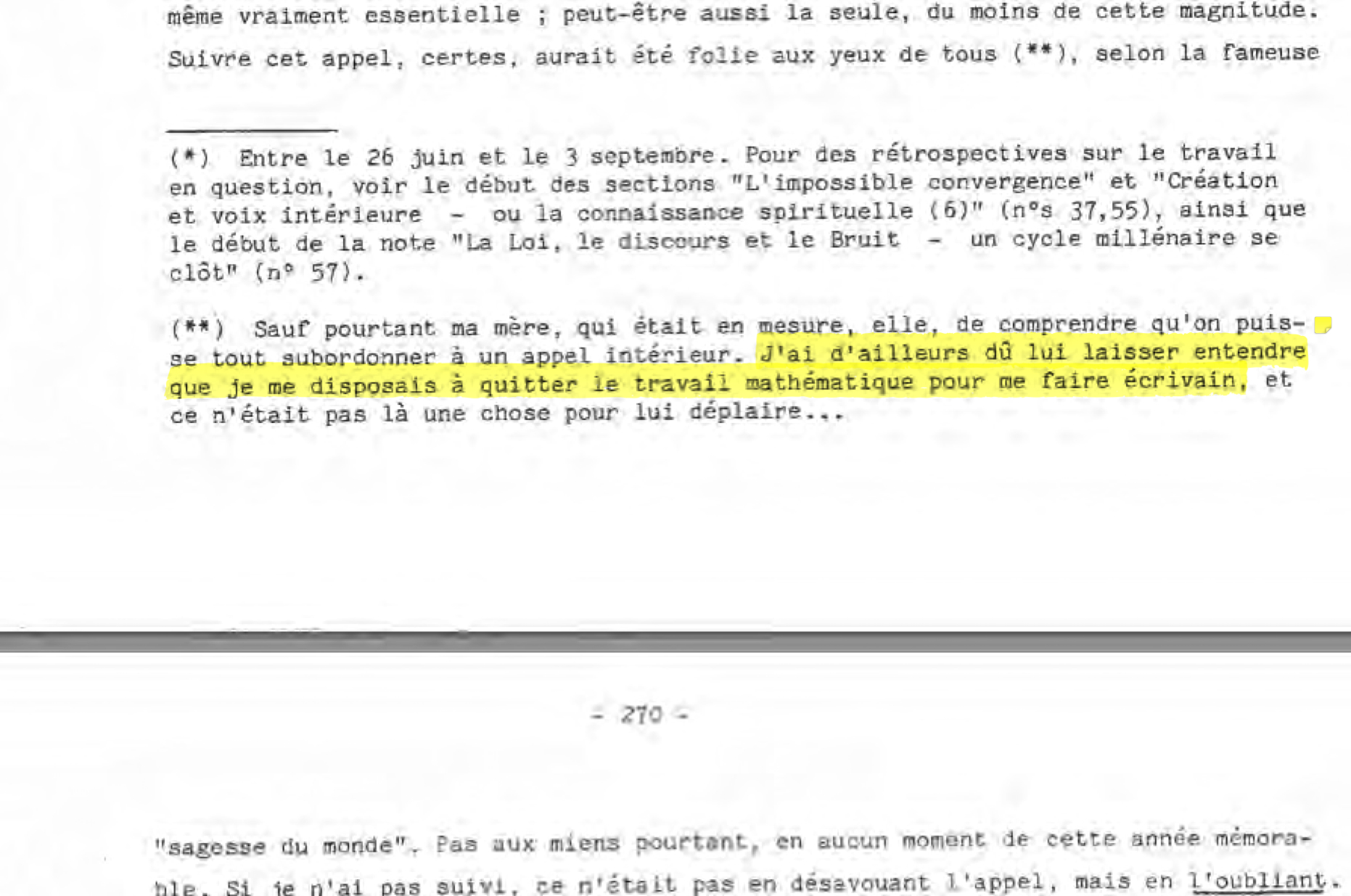Probably I’m reading way too much into it, but it appears to indicate that Grothendieck stopped doing mathematics to become … a writer!

April 23rd, 2014

Grothendieck documentary available on DVD

+catherine aira and Yves Le Pestipon made a 90 minute long documentary “Alexander Grothendieck, sur les routes d’un genie” which had successful showings in universities, at the Novela science festival, on Toulouse television, and elsewhere. It will be shown in Nantes, Toulouse, Montpellier, and Montreal.Yves Le Pestipon is one of the people behind the mysterious website lastree.net which has (among many other things) posts on Grothendieck containing hints to his present whereabouts…

clip1

clip2

Here’s the tumblr page of the project:

All of us who cannot attend the viewings can still order the DVD for 25 Euros (20 Euros in France) by sending an email to catherine.aira@gmail.com.

A new release of the DVD, containing English subtitles, will be available soon.

Thanks to +Adeel Khan Yusufzai +David Roberts and +catherine aira

“Pythagorean Crimes” by Tefcros Michaelides is a murder mystery set at the beginning of the 20th century. It starts with Hilbert’s address at the 1900 ICM in Paris (in which he gives his list of problems, such as the 2nd, his program for a finitistic proof of the consistency of the axioms of arithmetic) and ends in the early 1930ties (perhaps you can by now already guess which theorem will play a crucial role in the plot?).

It depicts beautifully daily (or better, nightly) life in mathematical and artistic circles, especially in Paris between 1900 and 1906.

Bricard, Caratheodory, Dedekind, Dehn, De la Vallee-Poussin, Frege, Godel, Hadamard, Hamel, Hatzidakis, Hermite, Hilbert, Klein, Lindemann, Minkowski, Peano, Poincare, Reynaud, Russell and Whitehead all make a brief appearance, as do Appollinaire, Casagemas, Cezanne, Degas, Derain, Max Jacob, Jacobides, Lumiere, Matisse, Melies, Pallares, Picasso, Renoir, Salmon, Toulouse-Lautrec, Utrillo, Zola.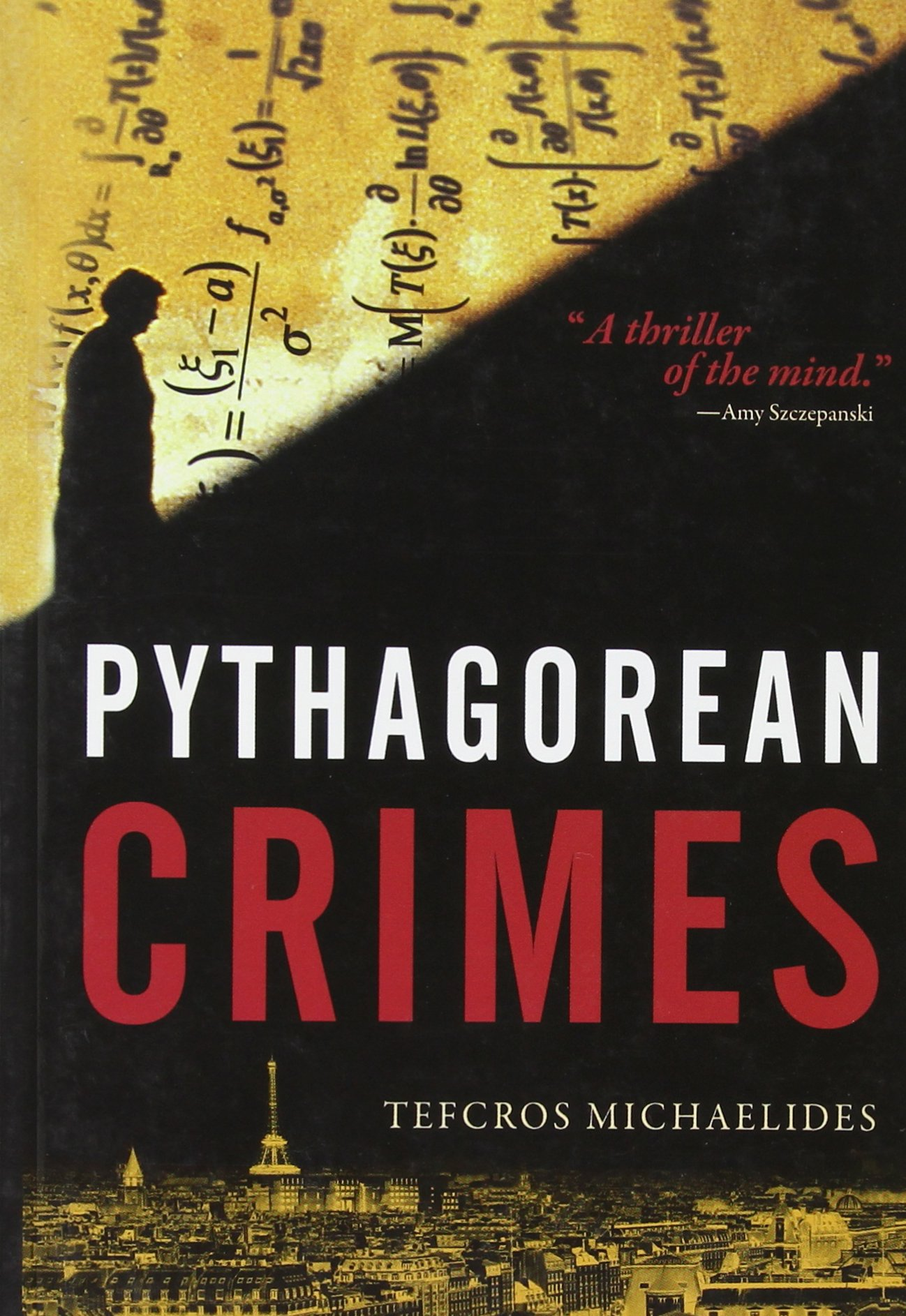Both lists contain names I had never heard of. But the biggest surprise, to me, was to discover the name of Maurice Princet, “le mathématicien du cubisme”.

Princet (1875-1973) was a mathematician who frequented the group around Pablo Picasso at the Bateau-Lavoir in Montmartre (at least until 1907 when his wife left him for the painter Derain).

Princet introduced the group to the works of Poincare and the concept of the 4-th dimension. He gave Picasso the book “Traité élémentaire de géométrie à quatre dimensions” by Jouffret, describing hyper-cubes and other polyhedra in 4 dimensions and ways to project them dowm to the 2 dimensions of the canvas.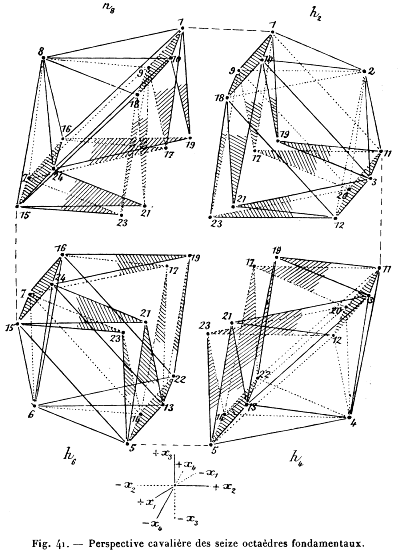This book appears to have been influential in the genesis of Picasso’s Les Demoiselles d’Avignon (the painting also appears, in an unfinished state, in “Pythagorean Crimes”).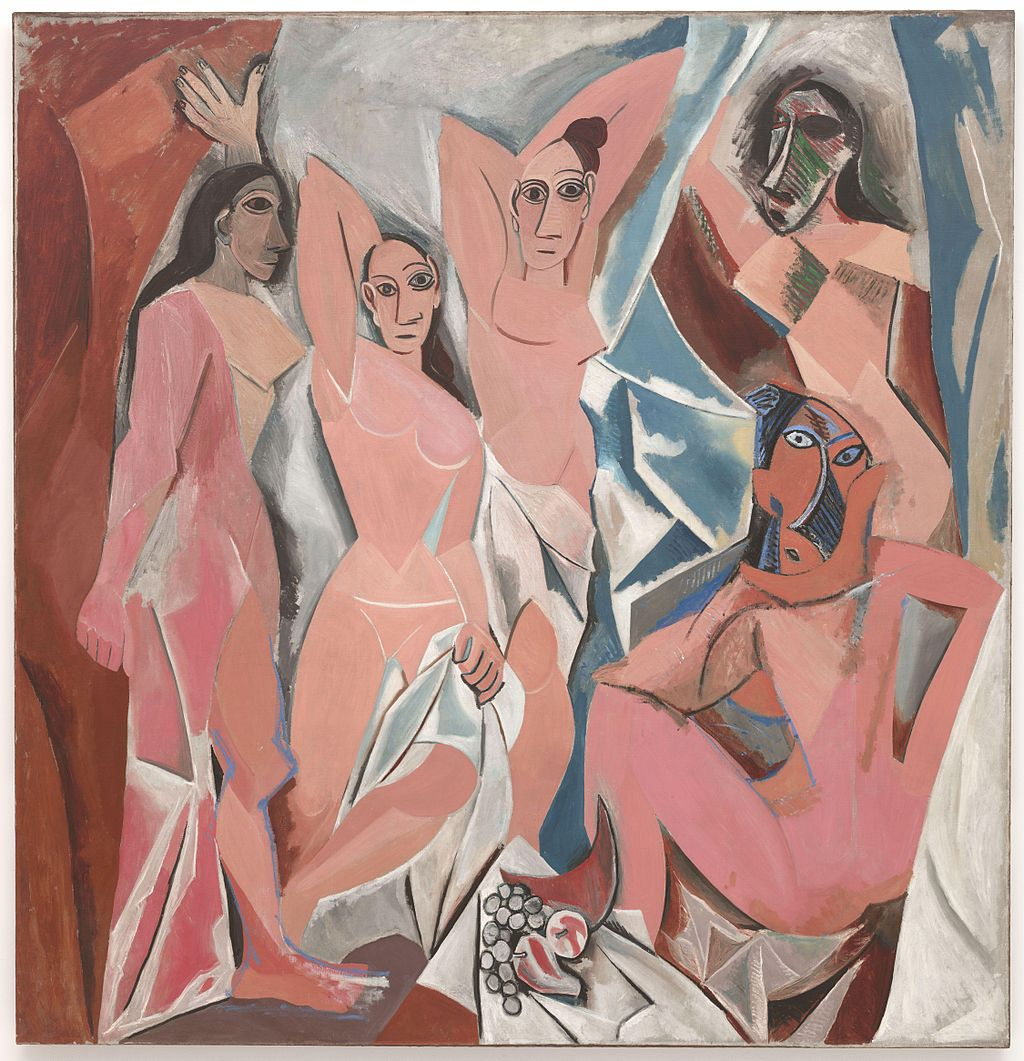Some other painters tried to capture movement with projections from the 4-th dimension. A nice example is Nude descending a staircase by Marcel Duchamp (mostly known for his urinoir…).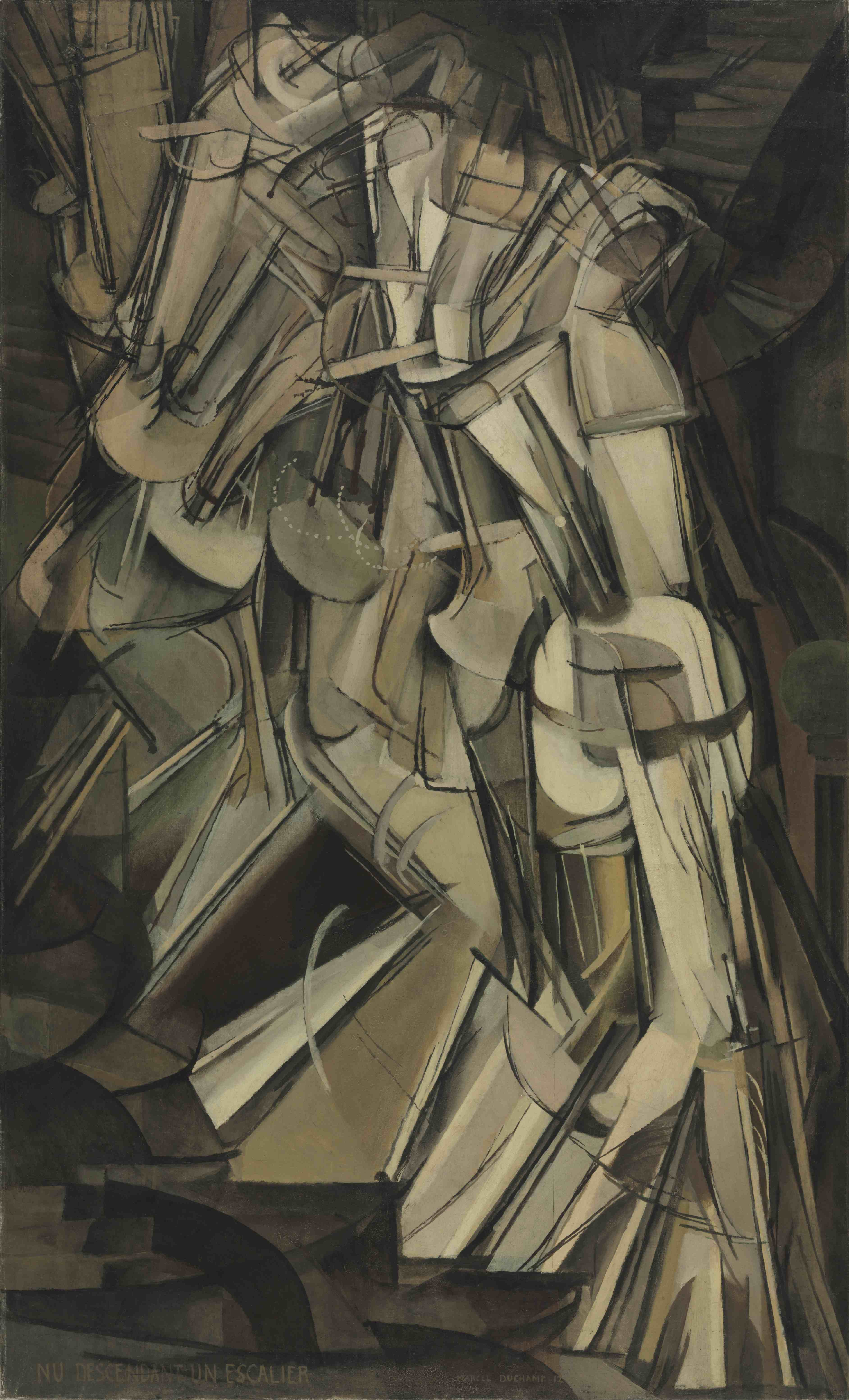Maurice Princet loved to get the artists interested in the new views on space. Duchamp told Pierre Cabanne, “We weren’t mathematicians at all, but we really did believe in Princet”.

I don’t know whether Duchamp liked Princet’s own attempts at painting. Here’s a cubistic work by Maurice Princet himself.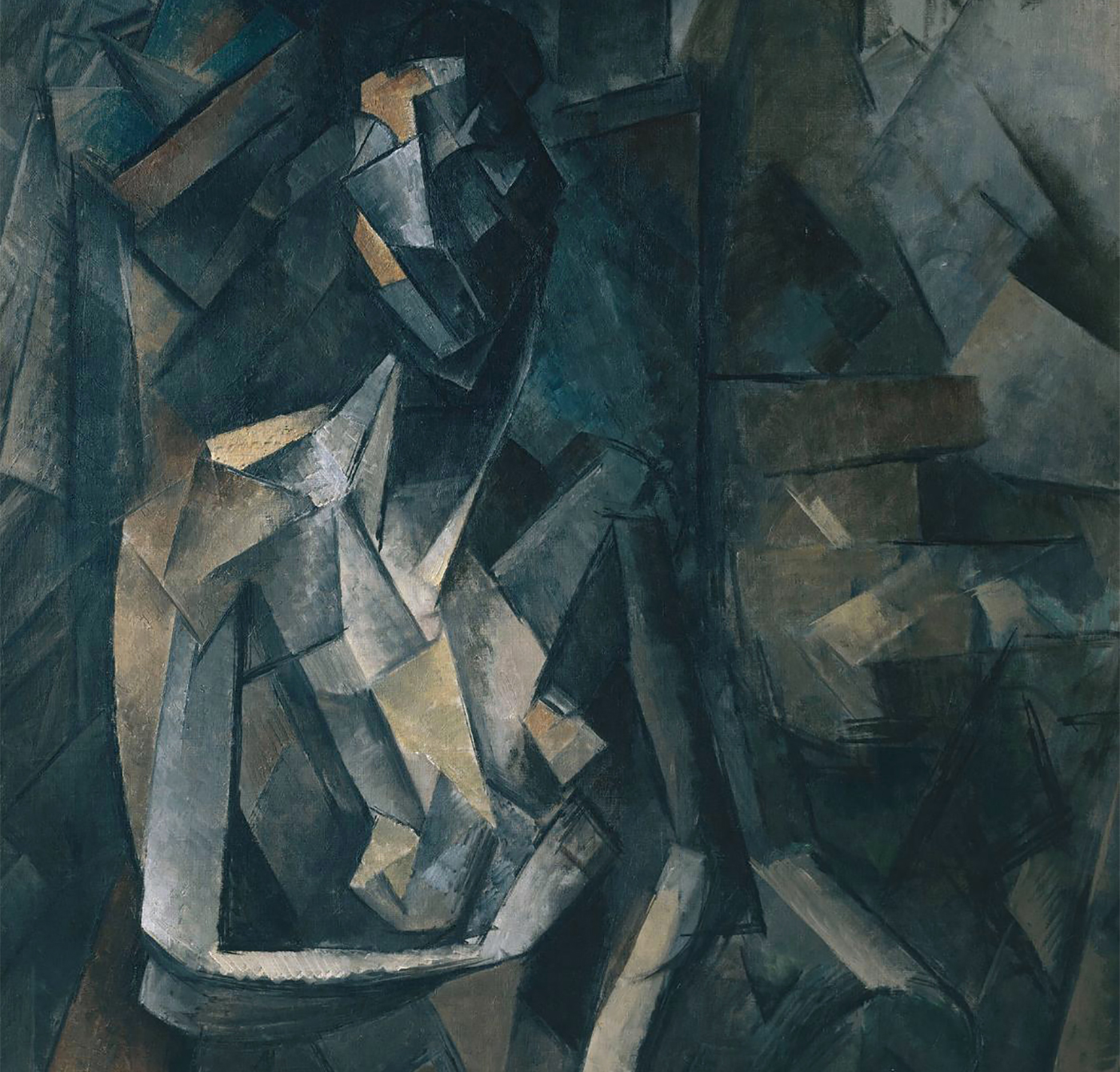The Fibonacci sequence reappears a bit later in Dan Brown’s book ‘The Da Vinci Code’ where it is used to login to the bank account of Jacques Sauniere at the fictitious Parisian branch of the Depository Bank of Zurich.

Last time we saw that the Hankel matrix of the Fibonacci series $F=(1,1,2,3,5,\dots)$ is invertible over $\mathbb{Z}$
$H(F) = \begin{bmatrix} 1 & 1 \\ 1 & 2 \end{bmatrix} \in SL_2(\mathbb{Z})$
and we can use the rule for the co-multiplication $\Delta$ on $\Re(\mathbb{Q})$, the algebra of rational linear recursive sequences, to determine $\Delta(F)$.

For a general integral linear recursive sequence the corresponding Hankel matrix is invertible over $\mathbb{Q}$, but rarely over $\mathbb{Z}$. So we need another approach to compute the co-multiplication on $\Re(\mathbb{Z})$.

Any integral sequence $a = (a_0,a_1,a_2,\dots)$ can be seen as defining a $\mathbb{Z}$-linear map $\lambda_a$ from the integral polynomial ring $\mathbb{Z}[x]$ to $\mathbb{Z}$ itself via the rule $\lambda_a(x^n) = a_n$.

If $a \in \Re(\mathbb{Z})$, then there is a monic polynomial with integral coefficients of a certain degree $n$

$f(x) = x^n + b_1 x^{n-1} + b_2 x^{n-2} + \dots + b_{n-1} x + b_n$

such that for every integer $m$ we have that

$a_{m+n} + b_1 a_{m+n-1} + b_2 a_{m+n-2} + \dots + b_{n-1} a_{m+1} + a_m = 0$

Alternatively, we can look at $a$ as defining a $\mathbb{Z}$-linear map $\lambda_a$ from the quotient ring $\mathbb{Z}[x]/(f(x))$ to $\mathbb{Z}$.

The multiplicative structure on $\mathbb{Z}[x]/(f(x))$ dualizes to a co-multiplication $\Delta_f$ on the set of all such linear maps $(\mathbb{Z}[x]/(f(x)))^{\ast}$ and we can compute $\Delta_f(a)$.

We see that the set of all integral linear recursive sequences can be identified with the direct limit
$\Re(\mathbb{Z}) = \underset{\underset{f|g}{\rightarrow}}{lim}~(\frac{\mathbb{Z}[x]}{(f(x))})^{\ast}$
(where the directed system is ordered via division of monic integral polynomials) and so is equipped with a co-multiplication $\Delta = \underset{\rightarrow}{lim}~\Delta_f$.

Btw. the ring structure on $\Re(\mathbb{Z}) \subset (\mathbb{Z}[x])^{\ast}$ comes from restricting to $\Re(\mathbb{Z})$ the dual structures of the co-ring structure on $\mathbb{Z}[x]$ given by
$\Delta(x) = x \otimes x \quad \text{and} \quad \epsilon(x) = 1$

From this description it is clear that you need to know a hell of a lot number theory to describe this co-multiplication explicitly.

As most of us prefer to work with rings rather than co-rings it is a good idea to begin to study this co-multiplication $\Delta$ by looking at the dual ring structure of
$\Re(\mathbb{Z})^{\ast} = \underset{\underset{ f | g}{\leftarrow}}{lim}~\frac{\mathbb{Z}[x]}{(f(x))}$
This is the completion of $\mathbb{Z}[x]$ at the multiplicative set of all monic integral polynomials.

This is a horrible ring and very little is known about it. Some general remarks were proved by Kazuo Habiro in his paper Cyclotomic completions of polynomial rings.

In fact, Habiro got interested is a certain subring of $\Re(\mathbb{Z})^{\ast}$ which we now know as the Habiro ring and which seems to be a red herring is all stuff about the field with one element, $\mathbb{F}_1$ (more on this another time). Habiro’s ring is

$\widehat{\mathbb{Z}[q]} = \underset{\underset{n|m}{\leftarrow}}{lim}~\frac{\mathbb{Z}[q]}{(q^n-1)}$

and its elements are all formal power series of the form
$a_0 + a_1 (q-1) + a_2 (q^2-1)(q-1) + \dots + a_n (q^n-1)(q^{n-1}-1) \dots (q-1) + \dots$
with all coefficients $a_n \in \mathbb{Z}$.

Here’s a funny property of such series. If you evaluate them at $q \in \mathbb{C}$ these series are likely to diverge almost everywhere, but they do converge in all roots of unity!

Some people say that these functions are ‘leaking out of the roots of unity’.

If the ring $\Re(\mathbb{Z})^{\ast}$ is controlled by the absolute Galois group $Gal(\overline{\mathbb{Q}}/\mathbb{Q})$, then Habiro’s ring is controlled by the abelianzation $Gal(\overline{\mathbb{Q}}/\mathbb{Q})^{ab} \simeq \hat{\mathbb{Z}}^{\ast}$.

The monstrous moonshine picture is the finite piece of Conway’s Big Picture needed to understand the 171 moonshine groups associated to conjugacy classes of the monster.

Last time I claimed that there were exactly 7 types of local behaviour, but I missed one. The forgotten type is centered at the number lattice $84$.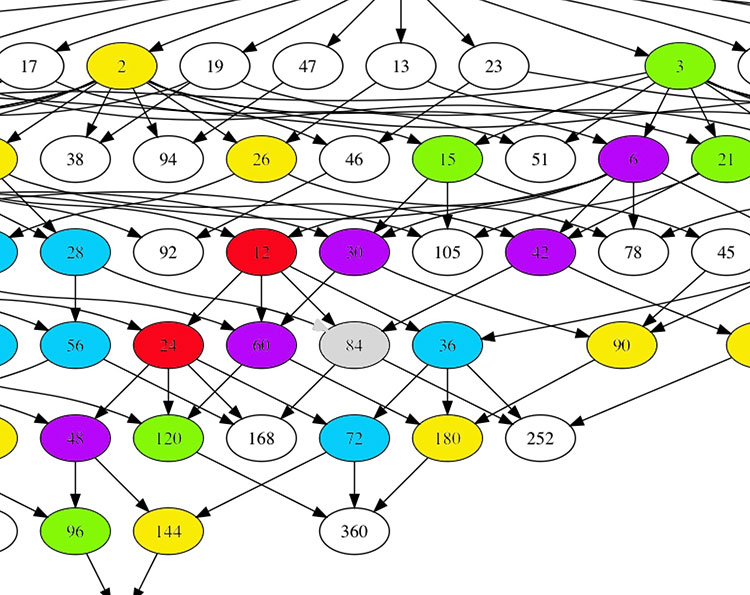Locally around it the moonshine picture looks like this
$\xymatrix{42 \ar@{-}[dr] & 28 \frac{1}{3} \ar@[red]@{-}[d] & 41 \frac{1}{2} \ar@{-}[ld] \\ 28 \ar@[red]@{-}[r] & \color{grey}{84} \ar@[red]@{-}[r] \ar@[red]@{-}[d] \ar@{-}[rd] & 28 \frac{2}{3} \\ & 252 & 168}$

and it involves all square roots of unity ($42$, $42 \frac{1}{2}$ and $168$) and $3$-rd roots of unity ($28$, $28 \frac{1}{3}$, $28 \frac{2}{3}$ and $252$) centered at $84$.

No, I’m not hallucinating, there are indeed $3$ square roots of unity and $4$ third roots of unity as they come in two families, depending on which of the two canonical forms to express a lattice is chosen.

In the ‘normal’ expression $M \frac{g}{h}$ the two square roots are $42$ and $42 \frac{1}{2}$ and the three third roots are $28, 28 \frac{1}{3}$ and $28 \frac{2}{3}$. But in the ‘other’ expression
$M \frac{g}{h} = (\frac{g’}{h},\frac{1}{h^2M})$
(with $g.g’ \equiv 1~mod~h$) the families of $2$-nd and $3$-rd roots of unity are
$\{ 42 \frac{1}{2} = (\frac{1}{2},\frac{1}{168}), 168 = (0,\frac{1}{168}) \}$
and
$\{ 28 \frac{1}{3} = (\frac{1}{3},\frac{1}{252}), 28 \frac{2}{3} = (\frac{2}{3},\frac{1}{252}), 252 = (0 , \frac{1}{252}) \}$
As in the tetrahedral snake post, it is best to view the four $3$-rd roots of unity centered at $84$ as the vertices of a tetrahedron with center of gravity at $84$. Power maps in the first family correspond to rotations along the axis through $252$ and power maps in the second family are rotations along the axis through $28$.

In the ‘normal’ expression of lattices there’s then a total of 8 different local types, but two of them consist of just one number lattice: in $8$ the local picture contains all square, $4$-th and $8$-th roots of unity centered at $8$, and in $84$ the square and $3$-rd roots.

Perhaps surprisingly, if we redo everything in the ‘other’ expression (and use the other families of roots of unity), then the moonshine picture has only 7 types of local behaviour. The forgotten type $84$ appears to split into two occurrences of other types (one with only square roots of unity, and one with only $3$-rd roots).

I wonder what all this has to do with the action of the Bost-Connes algebra on the big picture or with Plazas’ approach to moonshine via non-commutative geometry.

In The Da Vinci Code, Dan Brown feels he need to bring in a French cryptologist, Sophie Neveu, to explain the mystery behind this series of numbers:

13 – 3 – 2 – 21 – 1 – 1 – 8 – 5

The Fibonacci sequence, 1-1-2-3-5-8-13-21-34-55-89-144-… is such that any number in it is the sum of the two previous numbers.

It is the most famous of all integral linear recursive sequences, that is, a sequence of integers

$a = (a_0,a_1,a_2,a_3,\dots)$

such that there is a monic polynomial with integral coefficients of a certain degree $n$

$f(x) = x^n + b_1 x^{n-1} + b_2 x^{n-2} + \dots + b_{n-1} x + b_n$

such that for every integer $m$ we have that

$a_{m+n} + b_1 a_{m+n-1} + b_2 a_{m+n-2} + \dots + b_{n-1} a_{m+1} + a_m = 0$

For the Fibonacci series $F=(F_0,F_1,F_2,\dots)$, this polynomial can be taken to be $x^2-x-1$ because
$F_{m+2} = F_{m+1}+F_m$

The set of all integral linear recursive sequences, let’s call it $\Re(\mathbb{Z})$, is a beautiful object of great complexity.

For starters, it is a ring. That is, we can add and multiply such sequences. If

$a=(a_0,a_1,a_2,\dots),~\quad \text{and}~\quad a’=(a’_0,a’_1,a’_2,\dots)~\quad \in \Re(\mathbb{Z})$

then the sequences

$a+a’ = (a_0+a’_0,a_1+a’_1,a_2+a’_2,\dots) \quad \text{and} \quad a \times a’ = (a_0.a’_0,a_1.a’_1,a_2.a’_2,\dots)$

are again linear recursive. The zero and unit in this ring are the constant sequences $0=(0,0,\dots)$ and $1=(1,1,\dots)$.

So far, nothing terribly difficult or exciting.

It follows that $\Re(\mathbb{Z})$ has a co-unit, that is, a ring morphism

$\epsilon~:~\Re(\mathbb{Z}) \rightarrow \mathbb{Z}$

sending a sequence $a = (a_0,a_1,\dots)$ to its first entry $a_0$.

It’s a bit more difficult to see that $\Re(\mathbb{Z})$ also has a co-multiplication

$\Delta~:~\Re(\mathbb{Z}) \rightarrow \Re(\mathbb{Z}) \otimes_{\mathbb{Z}} \Re(\mathbb{Z})$
with properties dual to those of usual multiplication.

To describe this co-multiplication in general will have to await another post. For now, we will describe it on the easier ring $\Re(\mathbb{Q})$ of all rational linear recursive sequences.

For such a sequence $q = (q_0,q_1,q_2,\dots) \in \Re(\mathbb{Q})$ we consider its Hankel matrix. From the sequence $q$ we can form symmetric $k \times k$ matrices such that the opposite $i+1$-th diagonal consists of entries all equal to $q_i$
$H_k(q) = \begin{bmatrix} q_0 & q_1 & q_2 & \dots & q_{k-1} \\ q_1 & q_2 & & & q_k \\ q_2 & & & & q_{k+1} \\ \vdots & & & & \vdots \\ q_{k-1} & q_k & q_{k+1} & \dots & q_{2k-2} \end{bmatrix}$
The Hankel matrix of $q$, $H(q)$ is $H_k(q)$ where $k$ is maximal such that $det~H_k(q) \not= 0$, that is, $H_k(q) \in GL_k(\mathbb{Q})$.

Let $S(q)=(s_{ij})$ be the inverse of $H(q)$, then the co-multiplication map
$\Delta~:~\Re(\mathbb{Q}) \rightarrow \Re(\mathbb{Q}) \otimes \Re(\mathbb{Q})$
sends the sequence $q = (q_0,q_1,\dots)$ to
$\Delta(q) = \sum_{i,j=0}^{k-1} s_{ij} (D^i q) \otimes (D^j q)$
where $D$ is the shift operator on sequence
$D(a_0,a_1,a_2,\dots) = (a_1,a_2,\dots)$

If $a \in \Re(\mathbb{Z})$ is such that $H(a) \in GL_k(\mathbb{Z})$ then the same formula gives $\Delta(a)$ in $\Re(\mathbb{Z})$.

For the Fibonacci sequences $F$ the Hankel matrix is
$H(F) = \begin{bmatrix} 1 & 1 \\ 1& 2 \end{bmatrix} \in GL_2(\mathbb{Z}) \quad \text{with inverse} \quad S(F) = \begin{bmatrix} 2 & -1 \\ -1 & 1 \end{bmatrix}$
and therefore
$\Delta(F) = 2 F \otimes ~F – DF \otimes F – F \otimes DF + DF \otimes DF$
There’s a lot of number theoretic and Galois-information encoded into the co-multiplication on $\Re(\mathbb{Q})$.

To see this we will describe the co-multiplication on $\Re(\overline{\mathbb{Q}})$ where $\overline{\mathbb{Q}}$ is the field of all algebraic numbers. One can show that

$\Re(\overline{\mathbb{Q}}) \simeq (\overline{\mathbb{Q}}[ \overline{\mathbb{Q}}_{\times}^{\ast}] \otimes \overline{\mathbb{Q}}[d]) \oplus \sum_{i=0}^{\infty} \overline{\mathbb{Q}} S_i$

Here, $\overline{\mathbb{Q}}[ \overline{\mathbb{Q}}_{\times}^{\ast}]$ is the group-algebra of the multiplicative group of non-zero elements $x \in \overline{\mathbb{Q}}^{\ast}_{\times}$ and each $x$, which corresponds to the geometric sequence $x=(1,x,x^2,x^3,\dots)$, is a group-like element
$\Delta(x) = x \otimes x \quad \text{and} \quad \epsilon(x) = 1$

$\overline{\mathbb{Q}}[d]$ is the universal Lie algebra of the $1$-dimensional Lie algebra on the primitive element $d = (0,1,2,3,\dots)$, that is
$\Delta(d) = d \otimes 1 + 1 \otimes d \quad \text{and} \quad \epsilon(d) = 0$

Finally, the co-algebra maps on the elements $S_i$ are given by
$\Delta(S_i) = \sum_{j=0}^i S_j \otimes S_{i-j} \quad \text{and} \quad \epsilon(S_i) = \delta_{0i}$

That is, the co-multiplication on $\Re(\overline{\mathbb{Q}})$ is completely known. To deduce from it the co-multiplication on $\Re(\mathbb{Q})$ we have to consider the invariants under the action of the absolute Galois group $Gal(\overline{\mathbb{Q}}/\mathbb{Q})$ as
$\Re(\overline{\mathbb{Q}})^{Gal(\overline{\mathbb{Q}}/\mathbb{Q})} \simeq \Re(\mathbb{Q})$

Unlike the Fibonacci sequence, not every integral linear recursive sequence has an Hankel matrix with determinant $\pm 1$, so to determine the co-multiplication on $\Re(\mathbb{Z})$ is even a lot harder, as we will see another time.

Reference: Richard G. Larson, Earl J. Taft, ‘The algebraic structure of linearly recursive sequences under Hadamard product’